# Author a multiple choice question

A multiple choice question presents a list of correct and incorrect answers that a student can choose from as their response.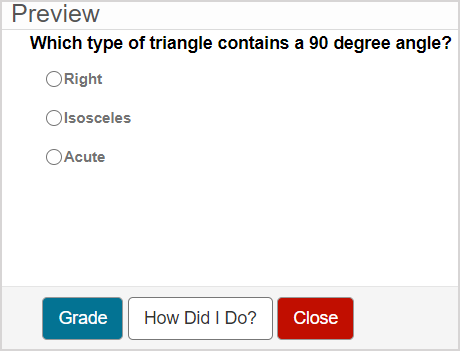You can define the following properties while authoring multiple choice questions:

• Whether the student must select a single answer choice as the correct answer or a set of answer choices as the correct answer
• The order of the answer choices
• The layout of the answer choices
• Feedback that's specific to each answer choice

NOTE: Partial grading is permitted for multiple choice questions when they're authored with a set of correct answers.

TIP: You can include an algorithm in your multiple choice question! Check out Author an algorithmic question.

TIP: All questions are authored using the Question Editor. Check out Author a question to learn how to access the Question Editor.

## To author a multiple choice question

To author a multiple choice question from the Question Editor:

1. Enter the question statement in the Question Text pane of the Question Editor.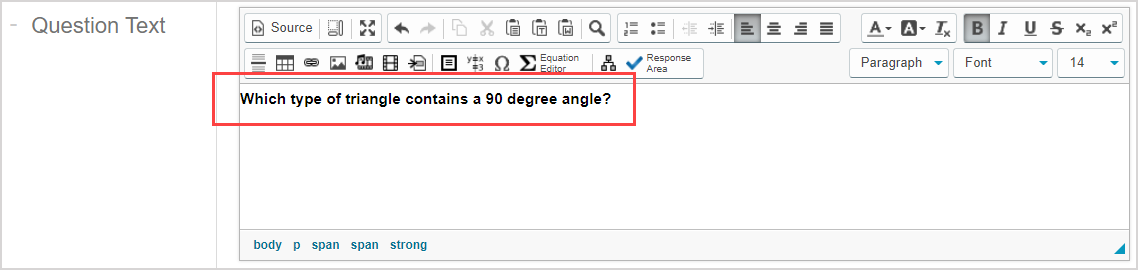TIP: Check out Use the Visual Editor for details on how to use this toolbar to the fullest to control the visual appearance of your matching question text.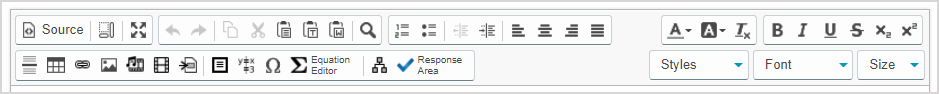1. Click Response Areato select the type of response area for this part of the question.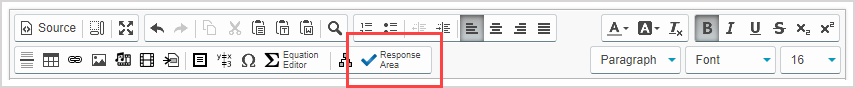NOTE: A question can have multiple question parts. Each question part have a different type of response area. Example — A question could have the first response area as a multiple choice response area, and a second response area as clickable image.

1. Click Multiple Choice from the list of response area types.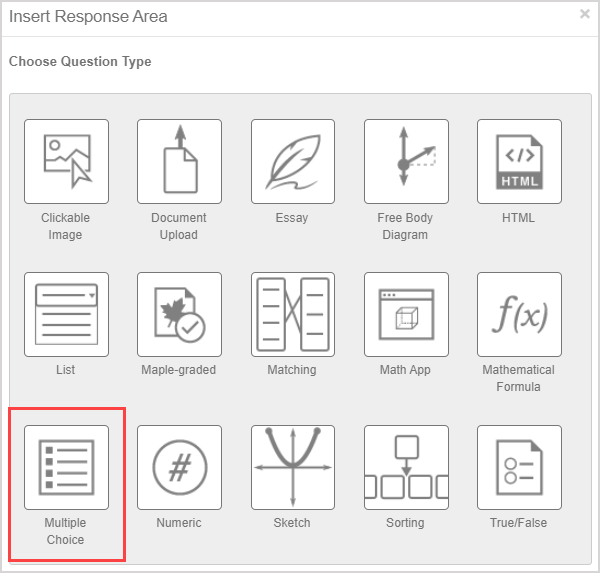1. Assign a weight to the response area. The default response area weighting is 1.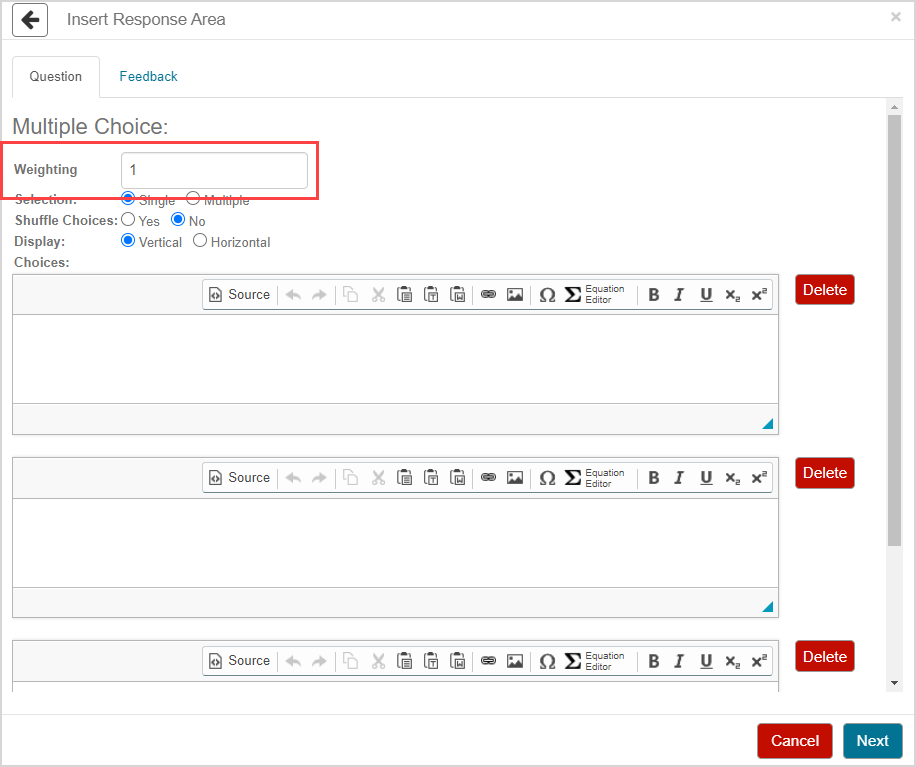TIP: The response area weight can be any integer greater than 0. Response area weighting is proportionate to the question total. Example — A question with two response areas could have the first response area weighted as 2, and the second response area weighted as 4. This means that the second response area will be worth twice as much as the first response area when calculating the total grade for the question.

1. Select how many correct answers your question will have using the Selection field:

• Single — There's only one correct answer for the question (selected by default).
• Multiple — There's more than one correct answer for the question.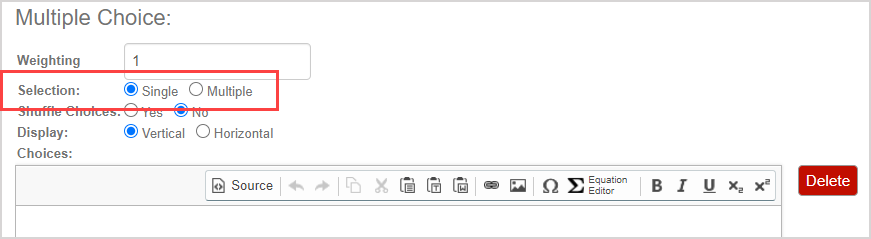TIP: Selecting Single will display radio button options for your answer choices implying that only one answer can be selected by the student.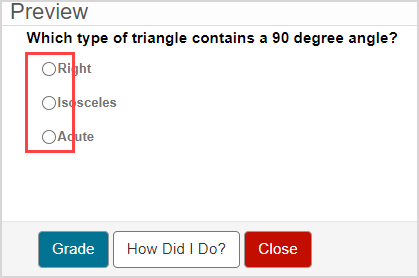Selecting Multiple will display check box options for your answer choices implying that multiple answers can be selected by the student.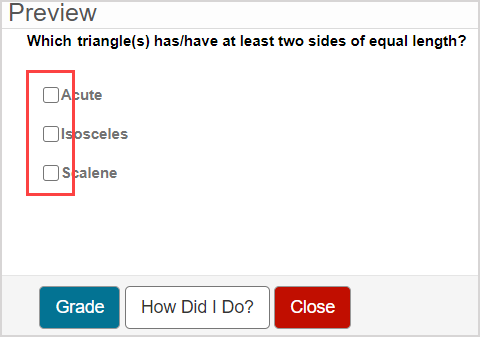Consider providing instructions to your students within your question text in case some students aren't aware of this functional difference.

1. Select how you want the order of answer choices to be displayed in the question using the Shuffle Choices field:

• Yes — The order of the answer choices is shuffled compared to the order that they were authored in.
• No — The order of the answer choices is displayed in the same order that they were authored in (selected by default).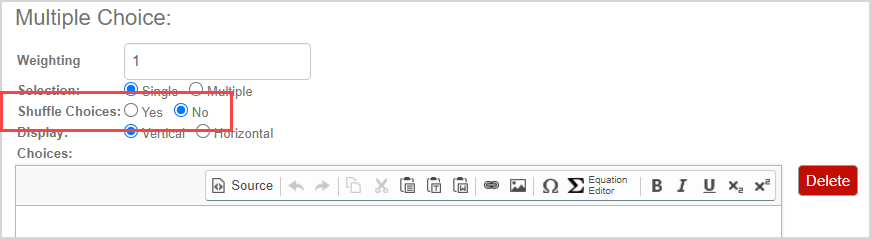1. Select the layout of how the answer choices are displayed in the question using the Display Choices field:

• Vertical — The answer choices are listed as a vertical list (selected by default).
• Horizontal — The answer choices are listed in a horizontal list.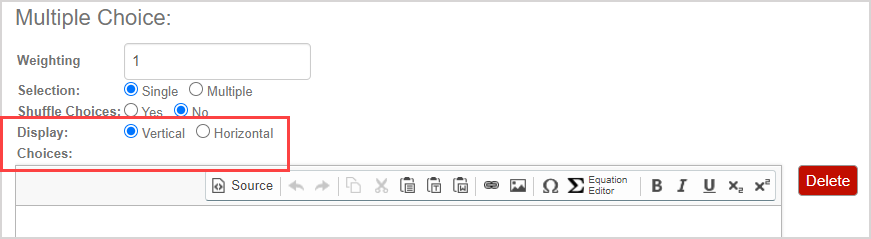1. Enter the details of each answer choice in each answer choice row.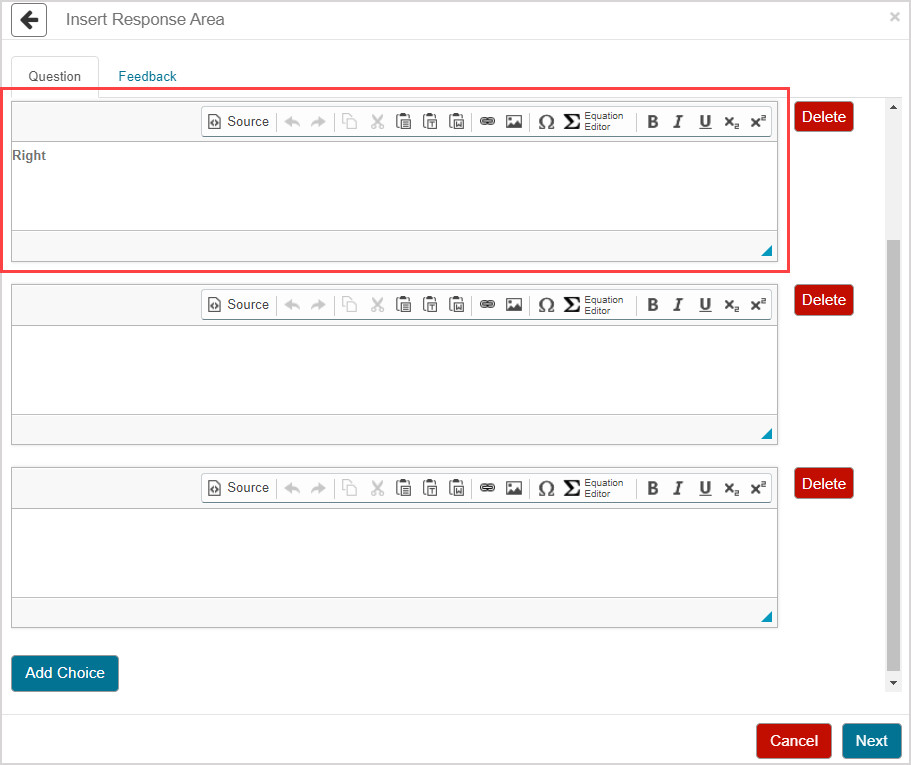TIP: Check out Use the Visual Editor for details on how to use this toolbar to the fullest to control the visual appearance of your answer choices.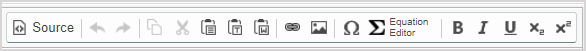TIP: Try modifying the question source code (check out Use the Source icon) or applying a theme (check out Work with themes) to modify the answer choice font size.

TIP: A multiple choice question starts with three answer choice rows by default. Your question must include at least two answer choices. You can click Add Choice as many times as needed to add more rows of answer choices.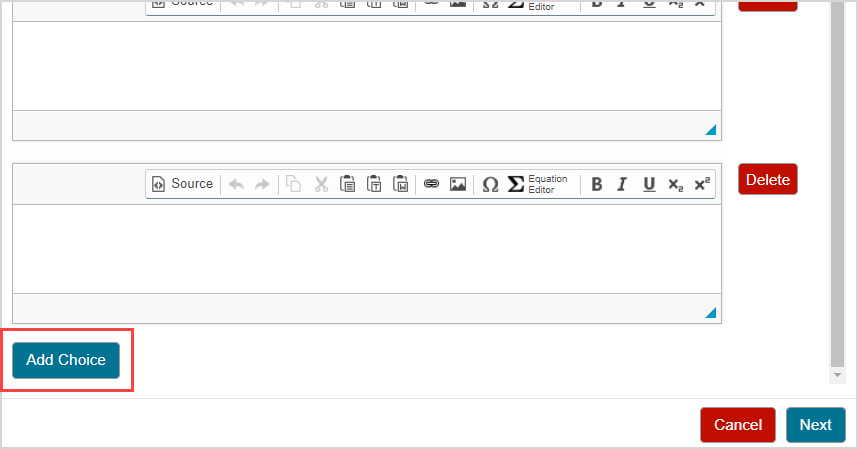Click Delete in the row of an answer choice to remove the row from your question.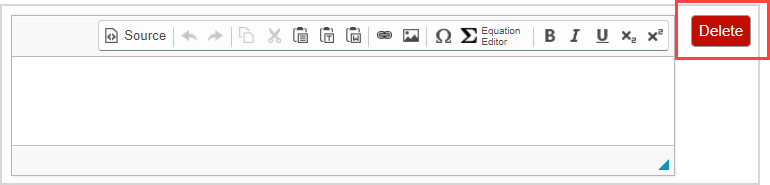1. Repeat step 8 as many times as needed and click Next after you've completed your answer choices.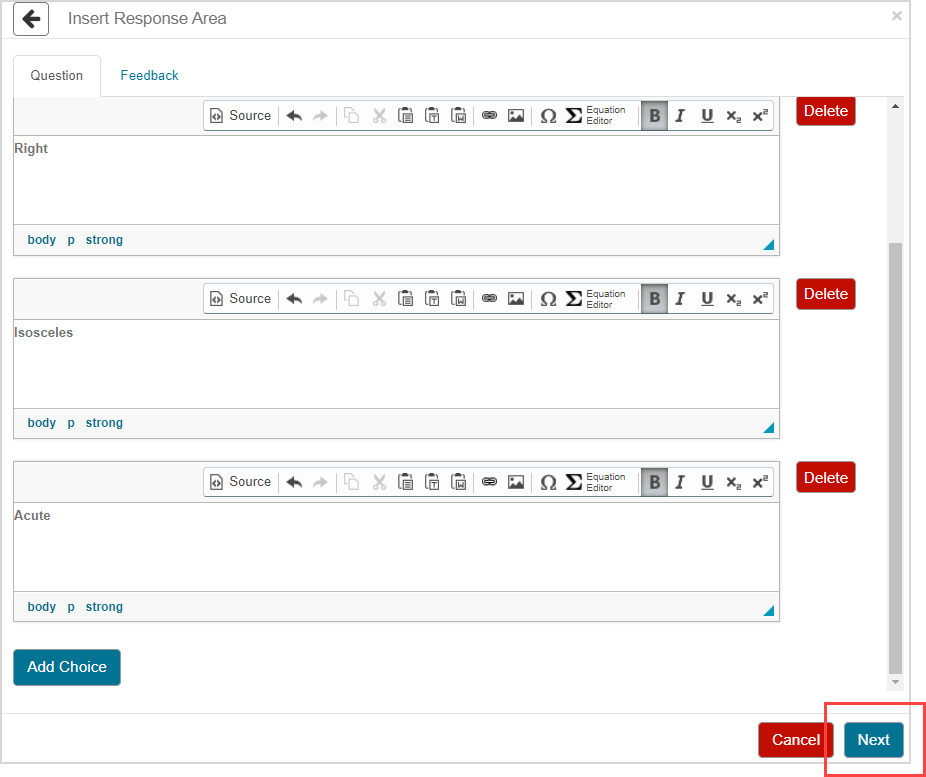1. Select the correct value(s) from the list of your answer choices:

• Select the correct value radio button if you selected Single at step 5.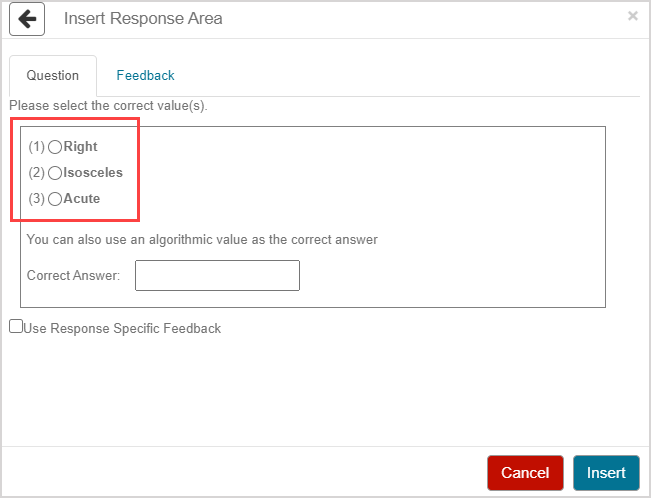• Select all of the correct value check boxes if you selected Multiple at step 5.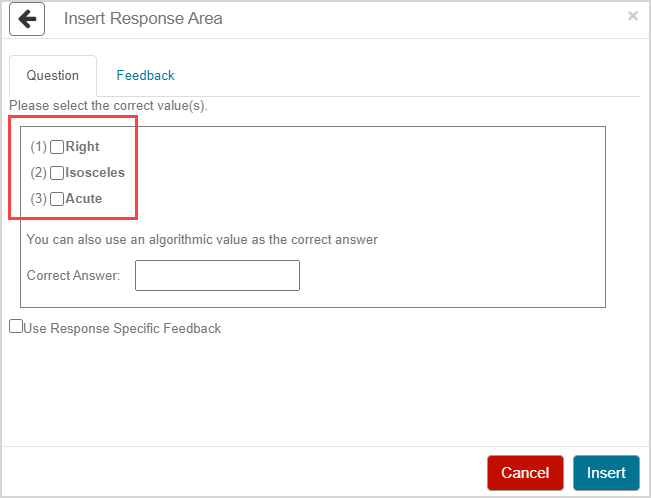TIP: Alternatively, you can use an algorithmic variable in the Correct Answer field.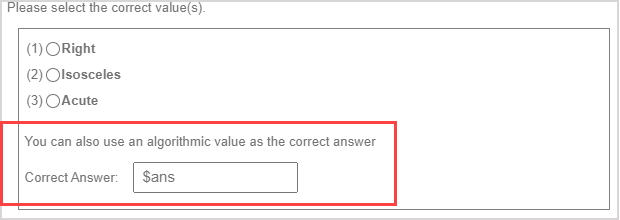The value of the algorithmic variable is calculated by the algorithm that you've defined in the Algorithm pane of the Question Editor. The calculated value indicates the correct answer choice(s). The calculated value must be:

• An integer between 1 and the total number of answer choices if you selected Single at step 5
• A list of integers separated by commas—each between 1 and the total number of answer choices—if you selected if you selected Multiple at step 5

Check out Author an algorithmic question for help with authoring an algorithm in the Algorithm pane of your multiple choice question.

1. (Optional) Select the Use Response Specific Feedback check box to author feedback that's specific to each answer choice.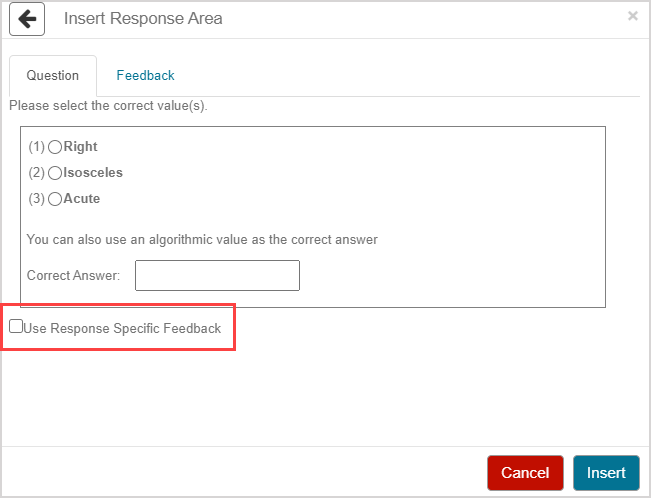1. (Optional) If you selected to use response specific feedback, author the feedback that's specific to each answer. A student will view this feedback if they submit that specific answer choice.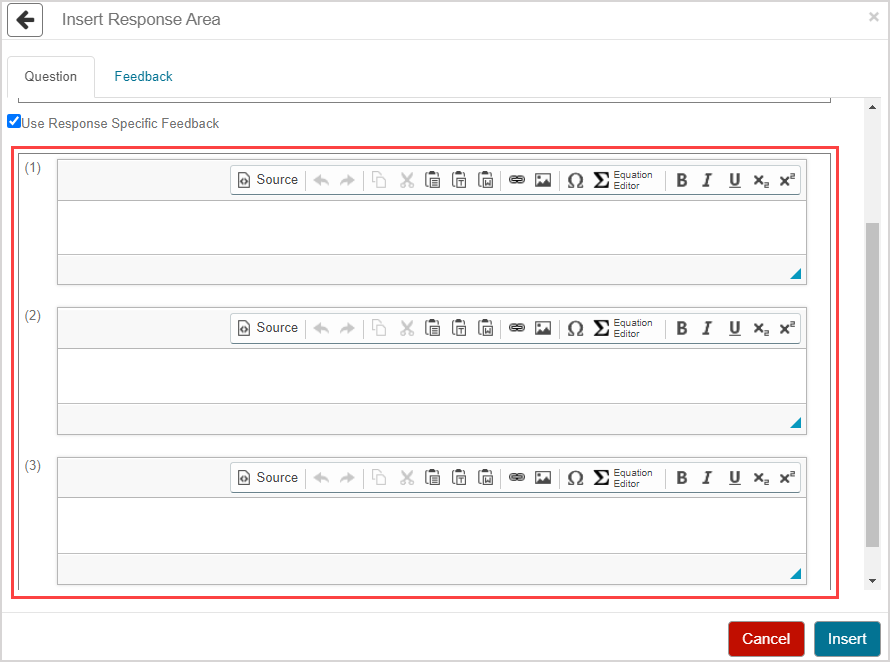TIP: Check out Use the Visual Editor for details on how to use this toolbar to the fullest to control the visual appearance of your response specific feedback.NOTE: If you deselect the Use Response Specific Feedback check box after authoring your feedback, a warning message is displayed stating that your authored feedback won't be saved. Click Close to continue.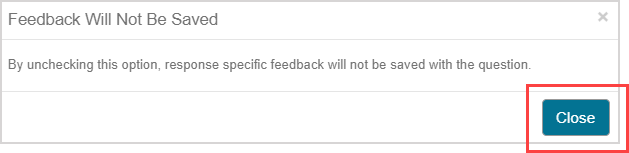1. Click Insert to add this response area to the question.1. The response area is inserted into your question.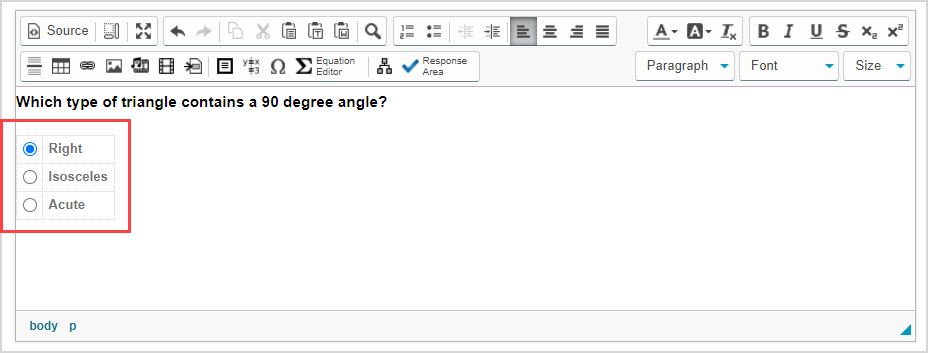1. Click Preview to trial your new question to ensure that it behaves as expected.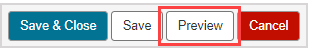TIP: You first have to click the save iconif you're creating this question from within an Activity Editor. After the question has been saved, you can then click the preview icon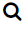to ensure that your new question behaves as expected.

1. Click Save to save your new question. Your new question is now stored in the Questions pane of the Content Repository.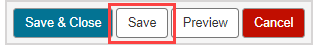TIP: Click the save iconif you're creating this question from within an Activity Editor. This saves your question to the activity and the Content Repository.

## Grading details for multiple choice questions

Multiple choice questions that are authored with one correct answer are graded as correct or incorrect; there's no partial grading allowed.

Multiple choice questions that are authored with a set of correct answers permit partial grading using the following calculation: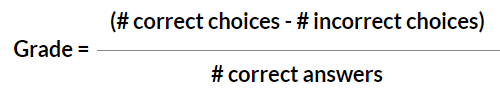The numerator will default to 0 if it's less than 0.

Example — A multiple choice question could have four answer choices, but only two of these are correct. If a student were to select only one of the two correct answers choices, their grade for the response area would be 50%: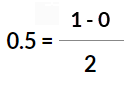If a student were to select one of the two correct answers choices and one incorrect answer choice, their grade for the response area would be 0%: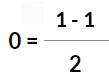NOTE: A student must select all of the correct answer choices for full marks if the question is authored with multiple correct answers.